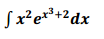Clutch Prep is now a part of Pearson

# The Substitution Rule

See all sections
Sections
Area
Indefinite Integrals
The Definite Integral
The Substitution Rule

Concept #1: Part 1: Inside of Parenthesis

Concept #2: Part 2: Denominators & Exponents

Concept #3: Part 3: Trigonometric Functions

Concept #4: Intro

Practice: Evaluate the following integral: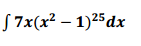Practice: Evaluate the following integral: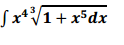Practice: Evaluate the following integral: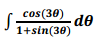Practice: Evaluate the following integral: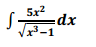Practice: Evaluate the following integral: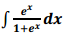Practice: Evaluate the following integral: